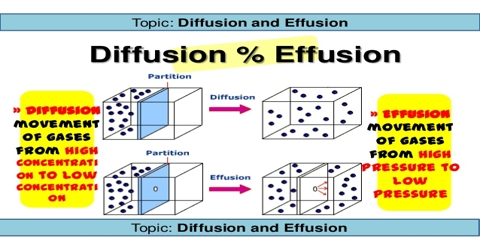# Diffusion and Effusion: Graham’s Law

Diffusion and Effusion: Graham’s Law of Diffusion

One characteristic of gases is that it mixes readily with other gases until the mixture is uniform. This mixing happens as a result of diffusion of one gas in to another.

Diffusion is the process in which one gas spreads out through another gas to occupy the space uniformly.

One can find diffusion in liquids also. If sugar is placed in a glass of unstirred water it will be found after some time that sugar distributes itself throughout the liquid and the mixture becomes uniformly sweet. As can be seen, sugar molecules have moved from a region of high concentration to a region where there was no sugar until the concentration becomes equal.

Graham found by experiment that gases diffuse through a porous diaphragm and that a. light gas diffuses more rapidly than a heavy gas. He described the diffusion of gases quantitatively in the form of a law which states that;

“The rate of diffusion of a gas at constant pressure and temperature is inversely proportional to the square root of its density”

This is known as ‘Graham’s Law of diffusion’.

A related phenomenon is the process in which a gas flows out of a container through small hole. This is called effusion. Experimentally it is simpler to measure effusion then diffusion. Graham studied the effusion of different gases through small holes and expressed his results in the form.

“The rate of effusion of gas molecules from a particular hole is inversely proportional to the square root of its density at constant temperature and pressure”

This is known as Graham’s law of effusion. Mathematically-

Rate of effusion ∞ (1/√ρ); here constant T and P.

or, Reffusion = k/√ρ; where k is constant.